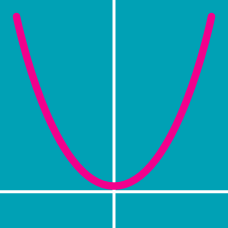Algebra

# Graphical Transformation - Problem Solving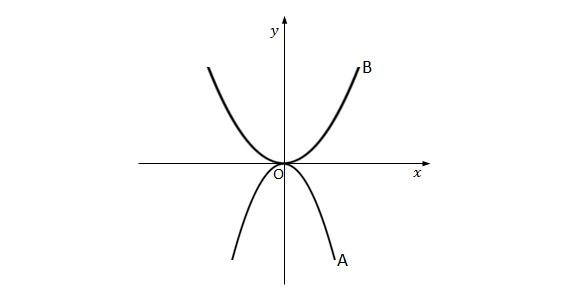In the above diagram, suppose the equation of curve A is $y=ax^2.$ Which of the following is a possible equation for curve B? \begin{aligned} \text{(i) } y&=5ax^2 &\qquad \text{(ii) } y&=-2ax^2 \\ \text{(iii) } y&=6ax^2 &\qquad \text{(iv) } y&=-\frac{1}{2}ax^2 \end{aligned}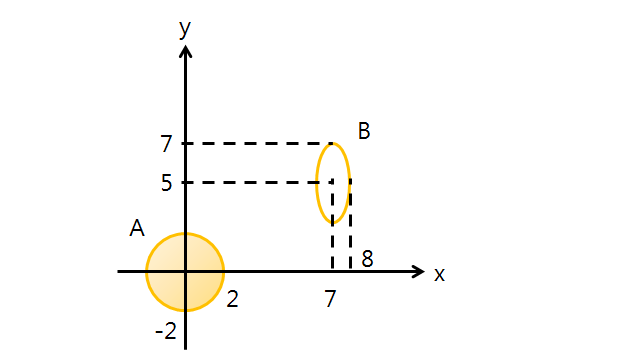In the above diagram, A is a circle with radius $2$ centered at the origin and B is an ellipse with major axis $4$ and minor axis $2$ centered at $(7, 5).$ If B is stretched and translated to obtain A, which of the following processes should be applied?

(i) Translate B by $-7$ and $-5$ in the positive directions of the $x$-axis and $y$-axis, respectively, and stretch by a factor of $2$ with respect to the $y$-axis.

(ii) Translate B by $-7$ and $-5$ in the positive directions of the $x$-axis and $y$-axis, respectively, and stretch by a factor of $2$ with respect to the $x$-axis.

(iii) Translate B by $7$ and $5$ in the positive directions of the $x$-axis and $y$-axis, respectively, and stretch by a factor of $\frac{1}{2}$ with respect to the $y$-axis.

(iv) Translate B by $-7$ and $-5$ in the positive directions of the $x$-axis and $y$-axis, respectively, and stretch by a factor of $\frac{1}{2}$ with respect to the $x$-axis.

Every point $(x,y)$ on the curve $y = \log_{2}{3x}$ is shifted to a new point by the translation $(x',y') =(x+m,y+n),$ where $m$ and $n$ are integers. The set of $(x',y')$ forms the curve $y = \log_{2}{(12 x-132)}$. What is the value of $m + n$?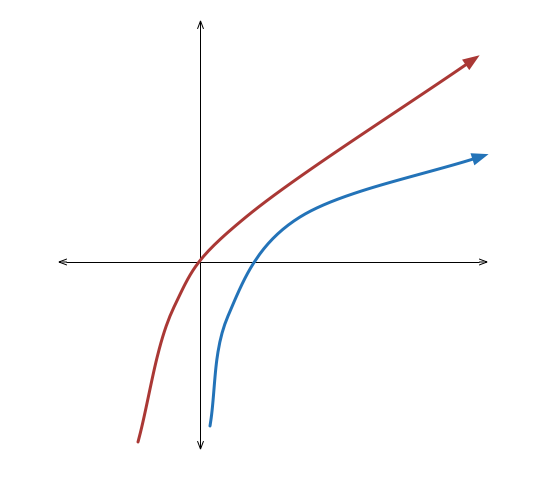Given the graph $y = \ln x$, which of these statements describes the transformations to get the graph of $y = \ln (4x^2 + 4x + 1)$ for $x > - \frac{1}{2}$?

$\quad \text{(1)}$ Translate to the left by 1 and up by $\ln 4$, then scale vertically by 2.

$\quad \text{(2)}$ Translate to the left by $\frac{1}{2}$ and up by $\ln 2$, then scale vertically by 2.

$\quad \text{(3)}$ Translate to the left by 1 and up by $\ln 2$, then scale vertically by 2.

$\quad \text{(4)}$ Translate to the left by $\frac{1}{2}$ and up by $\ln 4$, then scale vertically by 2.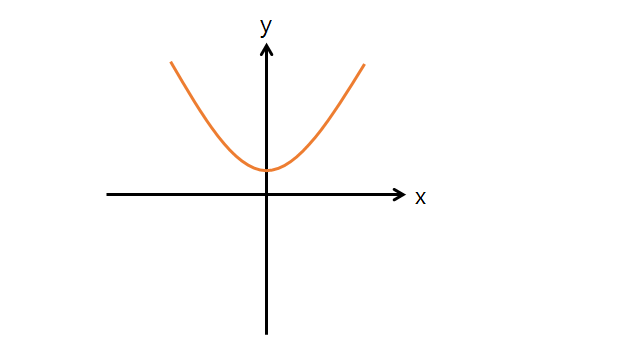Given the graph $y = 7x^2+7$, what is the sequence of operations required to obtain the graph of $y = 175x^2 +3?$

Note: The above graph is not drawn to scale.

(i) Stretch the given graph by a factor of $5$ with respect to the $x$-axis and translate by $4$ in the positive direction of the $x$-axis.

(ii) Stretch the given graph by a factor of $5$ with respect to the $y$-axis and translate by $-4$ in the positive direction of the $y$-axis.

(iii) Stretch the given graph by a factor of $\frac{1}{5}$ with respect to the $x$-axis and translate by $4$ in the positive direction of the $y$-axis.

(iv) Stretch the given graph by a factor of $\frac{1}{5}$ with respect to the $x$-axis and translate by $-4$ in the positive direction of the $y$-axis.

×